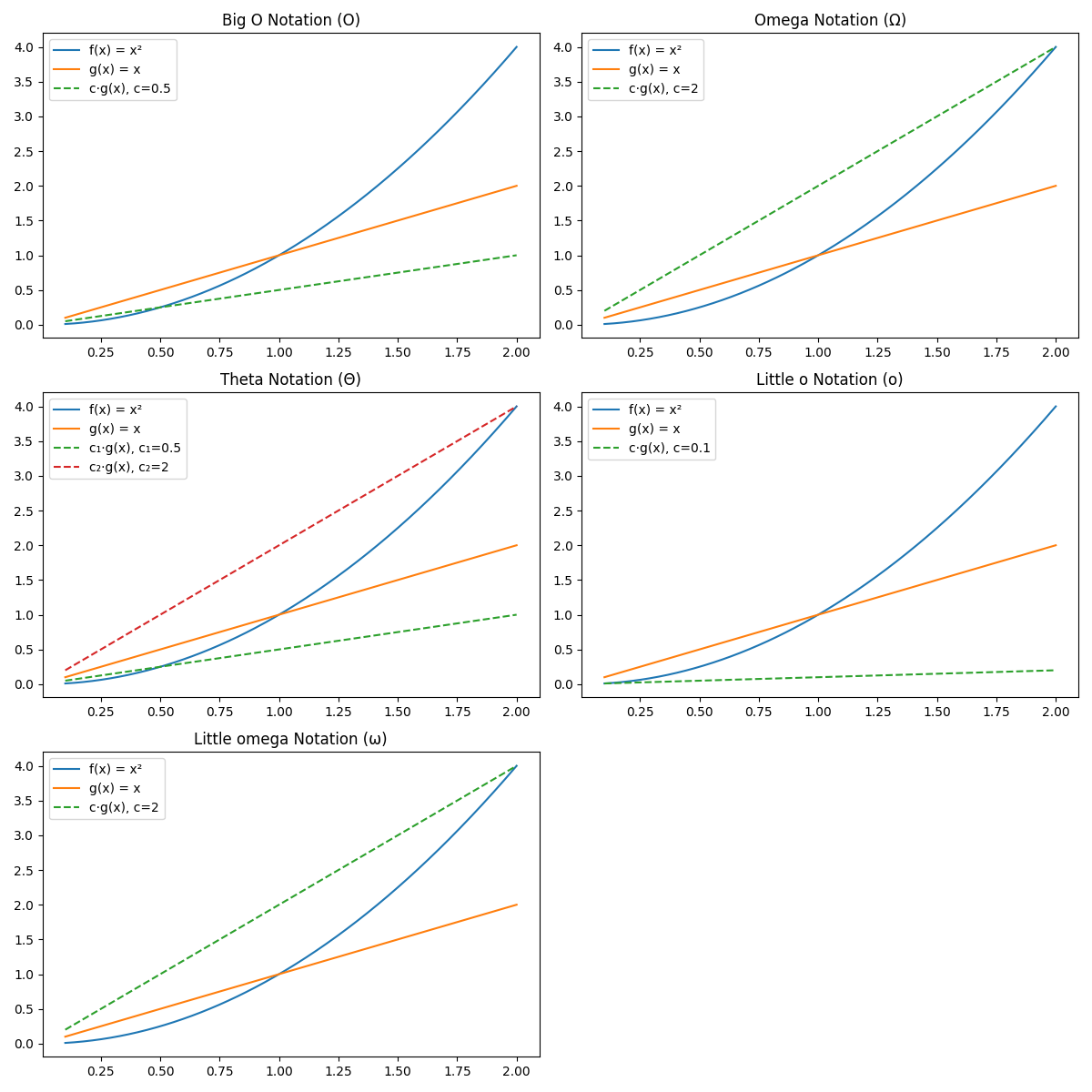# Complexity, Computation and Algorithms

Notes on Complexity theory, Theory of Computation and Algorithms

## Asymptotic Notations

Big O Notation [$O$]: This notation describes an upper bound on the growth rate of a function. It gives a worst-case scenario for the growth rate of an algorithm. Mathematically, we say that [$f(x) = O(g(x))$] if there exist constants [$c > 0$] and [$x_0 > 0$] such that [$f(x) \leq c \cdot g(x)$] for all [$x > x_0$].

Omega Notation [$\Omega$]: This notation describes a lower bound on the growth rate of a function. It gives a best-case scenario for the growth rate of an algorithm. Mathematically, [$f(x) = \Omega(g(x))$] if there exist constants [$c > 0$] and [$x_0 > 0$] such that [$f(x) \geq c \cdot g(x)$] for all [$x > x_0$].

Theta Notation [$\Theta$]: This notation describes both an upper and lower bound on the growth rate of a function, essentially bounding the function from above and below. Mathematically, [$f(x) = \Theta(g(x))$] if there exist constants [$c_1, c_2 > 0$] and [$x_0 > 0$] such that [$c_1 \cdot g(x) \leq f(x) \leq c_2 \cdot g(x)$] for all [$x > x_0$].

Little o Notation [$o$]: This notation describes an upper bound on the growth rate of a function but not a tight bound. It provides a notation for the behavior of a function as it approaches zero. Mathematically, [$f(x) = o(g(x))$] if for every constant [$c > 0$], there exists a [$x_0 > 0$] such that [$f(x) < c \cdot g(x)$] for all [$x > x_0$].

Little omega Notation [$\omega$]: This notation is the counterpart to little o notation but for lower bounds. It represents a lower bound but not a tight bound. Mathematically, [$f(x) = \omega(g(x))$] if for every constant [$c > 0$], there exists a [$x_0 > 0$] such that [$f(x) > c \cdot g(x)$] for all [$x > x_0$].Source: https://theoryofcomputation.xyz/blog/asymptotic-notations/, 0001-01-01